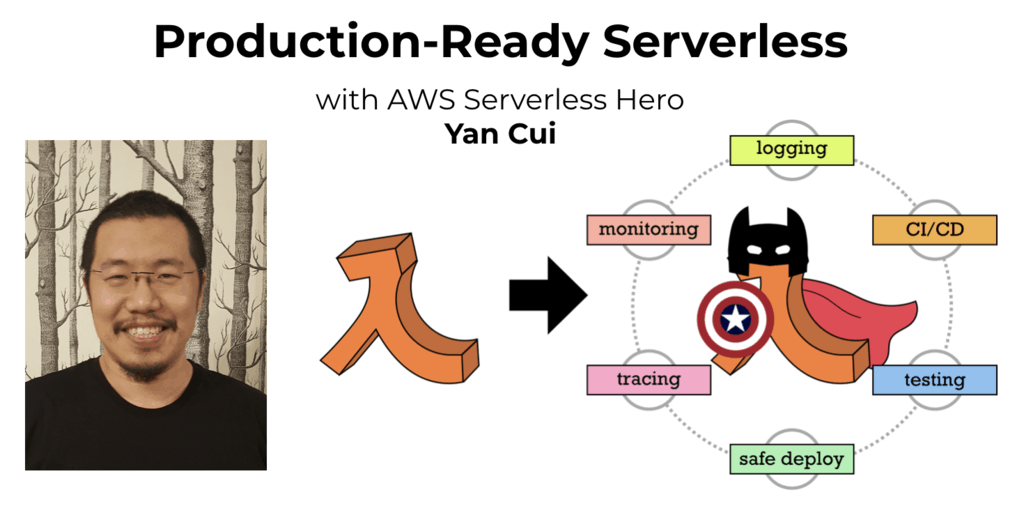# Equilibrium Index problem in F#

Check out my new course Learn you some Lambda best practice for great good! and learn the best practices for performance, cost, security, resilience, observability and scalability.

A good friend pointed me to a nice coding challenge she encountered – finding the Equilibrium Index of an array in O(n) time.

After a quick discussion we ended up with 2 solutions, and naturally I had to code them up in F### Solution 1

This is the same approach to the one you saw in the O(n) solution I posted the other day for the Multiply Others problem.

Here, we’ll create two temporary arrays – one that’s the running sum from the front, and the other the running sum from the rear, both are O(n) operations.

Once we have both, we can return all the indices where the front and rear arrays are equal.

(the questions usually only ask for one, but it’s nice to see all of them :-P)

This is a O(n) solution in both time and space.

## Solution 2

A more space efficient, O(1), solution is to:

• do one pass to sum the array (as the sum to the right)
• then starting from the front and iteratively subtract elements from that sum until you find an equilibrium index

for example, given the input array [ -1; 3; -4; 5; 1; -6; 2; 1 ], the sum is 1, so if we start from the front of the array:

• -1 : sum to the left is 0 (no elements), sum to the right is 1 – -1 = 2, no match
• 3 : sum to the left is -1, sum to the right is 2 – 3 = -1, match, so index 1 is an equilibrium index
• -4 : sum to the left is -1 + 3 = 2, sum to the right is -1 – -4 = 3, no match
• 5 : sum to the left is 2 + -4 = -2, sum to the right is 3 – 5 = -2, match, index 3 is also an equilibrium index
• 1 : sum to the left is -2 + 5 = 3, sum to the right is -2 – 1 = -3, no match
• -6 : sum to the left is 3 + 1 = 4, sum to the right is -3 – -6 = 3, no match
• 2 : sum to the left is 4 + -6 = -2, sum to the right is 3 – 2 = 1, no match
• 1 : sum to the left is -2 + 2 = 0, sum to the right is 1 – 1 = 0, match, index 7 is also an equilibrium index

So, applying this in F# I ended up with this:

notice that I’m generating the output array via comprehensions, this can look a bit odd to people new to F# so I tend to shy away from it and usually go for seq { … } |> Seq.toArray instead.

## Try it YourselfHi, I’m Yan. I’m an AWS Serverless Hero and the author of Production-Ready Serverless.

I specialise in rapidly transitioning teams to serverless and building production-ready services on AWS.

Are you struggling with serverless or need guidance on best practices? Do you want someone to review your architecture and help you avoid costly mistakes down the line? Whatever the case, I’m here to help.Check out my new course, Learn you some Lambda best practice for great good! In this course, you will learn best practices for working with AWS Lambda in terms of performance, cost, security, scalability, resilience and observability. Enrol now and enjoy a special preorder price of £9.99 (~\$13).Are you working with Serverless and looking for expert training to level-up your skills? Or are you looking for a solid foundation to start from? Look no further, register for my Production-Ready Serverless workshop to learn how to build production-grade Serverless applications!